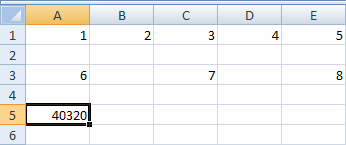# How to Multiply with Excel

In an earlier section, you saw how to multiply two numbers. You use the asterisk symbol between two cell references:

= A4 * B5

If you need to multiply more than two numbers, you don't have to do this:

= A4 * A5 * A6 * A7 * A8

You can use the colon (:) notation to shorten the formula. With addition, you used the word SUM, and placed your formula between round brackets:

= SUM(A4:A8)

With multiplication, you can use the word PRODUCT instead. Like this:

= PRODUCT(A4:A8)

The only thing that has changed here is the name of the inbuilt function: PRODUCT instead of SUM. But Excel 2007 will see the word Product and multiply whatever is between the round brackets.

You can use PRODUCT in the same way you did for SUM. For example, if you wanted to add up values in cells A4 to A8, and cells B4 and B5, you'd do it like this:

= PRODUCT(A4:A8, B4, B5)

To give you some practice, try these exercises.

Exercise
On a new worksheet, enter the number 1, 2, 3, 4 and 5. Put them into cells A1 to E1. Now use PRODUCT to multiply all five numbers. Place your answer in cell A3. If you get it right, your spreadsheet should look like ours:Exercise
For this exercise, delete your answer in cell A3. (You can do this by clicking into cell A3, and then hitting the Delete key on your keyboard). Now type a 6 in cell A3, a 7 in cell C3, and an 8 in cell E3. Use PRODUCT to multiply all 8 numbers. Place your answer in cell A5. Your spreadsheet will look like ours below, when you have the correct answer:In the next part, you'll see how to subtract and divide.

Subtract and Divide in Excel 2007 to 2016 -->

<--Back to the Excel Contents Page